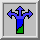# Monte Carlo Estimation for PiMonte Pi Java Applet

The Monte Carlo method is used in modeling a wide-range of physical systems at the forefront of scientific research today. Monte Carlo simulations are statistical models based on a series of random numbers.

Let's consider the problem of estimating Pi by utilizing the Monte Carlo method. Suppose you have a circle inscribed in a square (as in the figure). The experiment simply consists of throwing darts on this figure completely at random (meaning that every point on the dartboard has an equal chance of being hit by the dart). How can we use this experiment to estimate Pi?

The answer lies discovering the relationship between the geometry of the figure and the statistical outcome of throwing the darts.

Let's first look at the geometry of the figure.Let's assume the radius of the circle is R, then the Area of the circle = Pi R 2 and the Area of the square = 4 R2.

Now if we divide the area of the circle by the area of the square we get Pi / 4.

But, how do we estimate Pi by simulation? In the simulation, you keep throwing darts at random onto the dartboard. All of the darts fall within the square, but not all of them fall within the circle. Here's the key. If you throw darts completely at random, this experiment estimate the ratio of the area of the circle to the area of the square, by counting the number of darts in each.

Our study of the geometry tells us this ratio is Pi/4. So, now we can estimate Pi as

Pi = 4 x (Number of Darts in Circle) / (Number of Darts in Square)

Do you believe that the Monte Carlo simulation can indeed estimate Pi? Experiment with the MontePi Java applet.

Now that you've had a chance to experiment, can you answer these questions?

• Can you describe in words how the number of darts thrown effects the accuracy of the estimate?

• Make an average of, say, 5 trials of the estimate of Pi after 100, 1000 darts, 10000, 20000. Plot the average versus number of darts. Does it make a straight line?